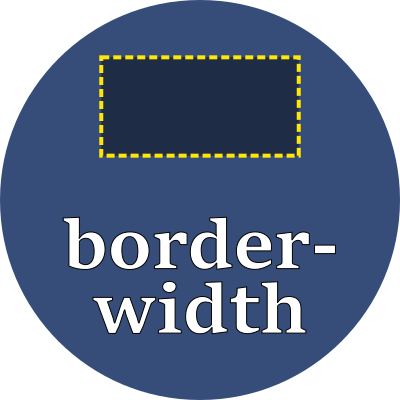# border-widthSet the thickness of an element's border.

## Property values

This property is used to set each of these four border width properties, which may also be set one by one:

This property expects anywhere from one to four values, with their interpretation as follows:

number of values sides effected
1 value Sets all four sides: top, right, bottom, left
2 values 1st value: top and bottom edges
2nd value: right and left edges
3 values 1st value: top edge
2nd value: right and left edges
3rd value: bottom edge
4 values 1st value: top edge
2nd value: right edge
3rd value: bottom edge
4th value: left edge

Use any of these font-relative typographic units to set the width:

 em em The `font-size` of the current font rem relative em The `font-size` of the element lh line height The `line-height` of the current font rlh relative line height The `line-height` of the element cap cap height The nominal height of the current font's capital letters ex "x" The height of the current font's "x" glyph ch "0" The advance measure (the width) of the current font's "0" glyph ic ideograph count The advance measure (the width) of the current font's "水" ideograph

Or use any of these fixed size units which are independent of the current font:

 in inch A size equal 96px px pixels A size equal to 1/96 inch pt point A size equal 1in/72 = 1.33px pc pica A size equal 1in/6 = 12pt = 16px cm centimeter A size equal to 96px/2.54 = 37.8px mm millimeter A size equal to 96px/25.4 = 3.8px Q quarter millimeter A size equal to 96px/1016 = 0.94px

## Examples

style > framing > border-widthBorder thickness